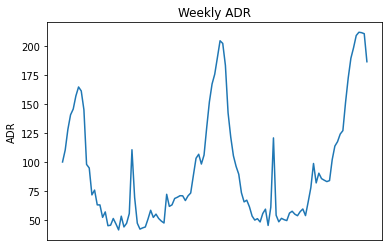# One-Step Predictions with LSTM: Forecasting Hotel RevenuesGenerating one-step predictions using LSTM. Note: This is an update to my previous article Forecasting Average Daily Rate Trends for Hotels Using LSTM. I since recognised a couple of technical errors in the original analysis, and decided to write a new article to address these and expand my prior analysis.

Note: This is an update to my previous article [Forecasting Average Daily Rate Trends for Hotels Using LSTM_](https://towardsdatascience.com/forecasting-average-daily-rate-trends-for-hotels-using-lstm-93a31e01190a). I since recognised a couple of technical errors in the original analysis, and decided to write a new article to address these and expand my prior analysis._

## Background

The purpose of using an LSTM model in this instance is to forecast ADR (average daily rate) for a hotel.

``ADR = Revenue ÷ sold rooms``

In this example, the average ADR for customers per week is calculated and formulated into a time series. The LSTM model is then used to forecast this metric on a week-by-week basis.

The original study by Antonio, Almeida and Nunes (2016) can be found here.

Using pandas, the average ADR is calculated per week. Here is a plot of the weekly ADR trend.Source: Jupyter Notebook Output

Note that the Jupyter Notebook for this example is available at the end of this article.

## Data Preparation

### 1. Normalizing data with MinMaxScaler

As with any neural network, the data needs to be scaled for proper interpretation by the network, a process known as normalization. MinMaxScaler is used for this purpose.

However, this comes with a caveat. Scaling must be done *after *the data has been split into training, validation and test sets — with each being scaled separately. A common mistake when first using the LSTM (I made this mistake myself) is to first normalize the data before splitting the data.

The reason this is erroneous is that the normalization technique will use data from the validation and test sets as a reference point when scaling the data as a whole. This will inadvertently influence the values of the training data, essentially resulting in data leakage from the validation and test sets.

In this regard, 100 data points are split into training and validation sets, with the last 15 data points being held as test data for comparison with the LSTM predictions.

## How to use Deep Learning for Time Series Forecasting

How to use Deep Learning for Time Series Forecasting. An application of the RNN family

## What is Time Series Forecasting?

In this article, we will be discussing an algorithm that helps us analyze past trends and lets us focus on what is to unfold next so this algorithm is time series forecasting. In this analysis, you have one variable -TIME. A time series is a set of observations taken at a specified time usually equal in intervals. It is used to predict future value based on previously observed data points.

## Most popular Data Science and Machine Learning courses — July 2020

Most popular Data Science and Machine Learning courses — August 2020. This list was last updated in August 2020 — and will be updated regularly so as to keep it relevant

## Forecasting Air Passenger Numbers with TensorFlow Probability

Forecasting air passenger numbers during COVID-19. TensorFlow Probability uses structural time series models to conduct time series forecasting.

## 15 Machine Learning and Data Science Project Ideas with Datasets

Learning is a new fun in the field of Machine Learning and Data Science. In this article, we’ll be discussing 15 machine learning and data science projects.